# Optical Isomerism

Optical isomerism is basically a type of stereoisomerism. Now, before we learn about optical isomerism let us quickly recall what are isomers and stereoisomers. Isomers are those compounds which have the same molecular formula but different bonding arrangement among atoms.

Whereas, in stereoisomer, both molecular formula and bonding arrangement of atoms are the same. However, they have different spatial (three dimensional) arrangement of atoms. It eliminates all different arrangements that are simply due to the molecule spinning in its entirety or revolving around unique bonds.

## What is Optical isomerism?

Optical isomerism is a case where the isomers display identical characteristics in terms of molecular weight as well as chemical and physical properties. However, they differ in their effect on the rotation of polarized light.

Optical isomerism occurs mainly in substances that have the same molecular and structural formula, but they cannot be superimposed on each other. In simple words, we can say that they are mirror images of each other. Alternatively, it can also be found in substances that have an asymmetric carbon atom.

Typically, optical isomerism is shown by stereoisomers which rotate the plane of polarized light. If the plane of polarized light passing through enantiomer solution rotates in the clockwise direction then the enantiomer is said to exist as (+) form and if the plane of polarized light rotates in anti-clockwise direction then the enantiomer is said to exist in (-).

Also Read: Isomers, Isomerism, Structural Isomerism

For example, an enantiomer of alanine (amino acid) which rotates the plane of polarized light in clockwise and anti-clockwise direction can be written as (+) alanine and. (-) alanine respectively.

The extent of rotation of plane-polarized light by the two enantiomeric form is exactly the same but the direction of rotation is opposite. Moreover, if the two enantiomer pair are present in equal amount then the resultant mixture is called a racemic mixture. This means that 50% of the mixture exists in (+) form and the other 50% exist in (-) form.

Since racemic mixture rotates the plane of polarized light equally in the opposite direction, the net rotation is zero. Therefore racemic mixture is optically inactive.

## Origin of Optical Isomers

To determine whether the compound is optically active or not, we have to first see whether the carbon is attached to four different groups or not. For a better understanding of optical isomerism, let us take an example of two models of organic compound as shown below;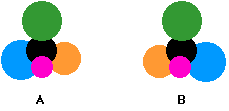These two models have the same bonding arrangement of the atom but a different spatial arrangement. From the above model of A and B, it is clear that the arrangement of the blue and orange group in space is different. Is it possible to align model A exactly like model B by rotating it? The answer is no. The reason for that is if we rotate A the arrangement of other group gets disturbed as shown below;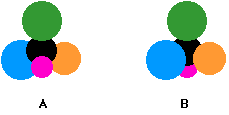We cannot make the spatial arrangement of A and B exactly the same by rotating them in any direction. A and B is said to be non-superimposable because we cannot make them look exactly.

Let us now see what will happen if a molecule containing two same groups attached to a central carbon atom is rotated as shown in the figure below;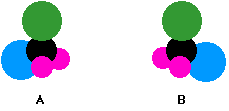Rotating molecule A by 180 degrees will give the same arrangement of the atom as that of B as shown below;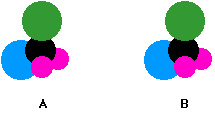From the above explanation, we can conclude that the compound will be optically active only if all the group attached to the central carbon atom are different.

## Chiral And Achiral Molecules

The difference between chiral and achiral molecules can be explained on the basis of the plane of symmetry. If all the attached group to the central carbon atom are different then there is no plane of symmetry. Such a molecule is known as a chiral molecule.

If all the group attached to the central carbon atom are not different then there exist plane of symmetry. Such molecules are called achiral molecules. It is clear that only molecule having chiral centre will show optical isomerism.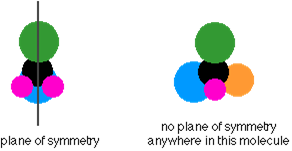## Relationship Between The Enantiomers

Enantiomers are a type of stereoisomers in which two molecules are a non-superimposable mirror image of each other.

In other words, one of the enantiomers is a mirror image of the other which cannot be superimposed. In other words, if a mirror looks at one isomer, it would see the other. The two isomers (the original and its mirror image) have a different spatial arrangement.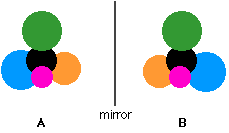Test Your Knowledge On Optical Isomerism!